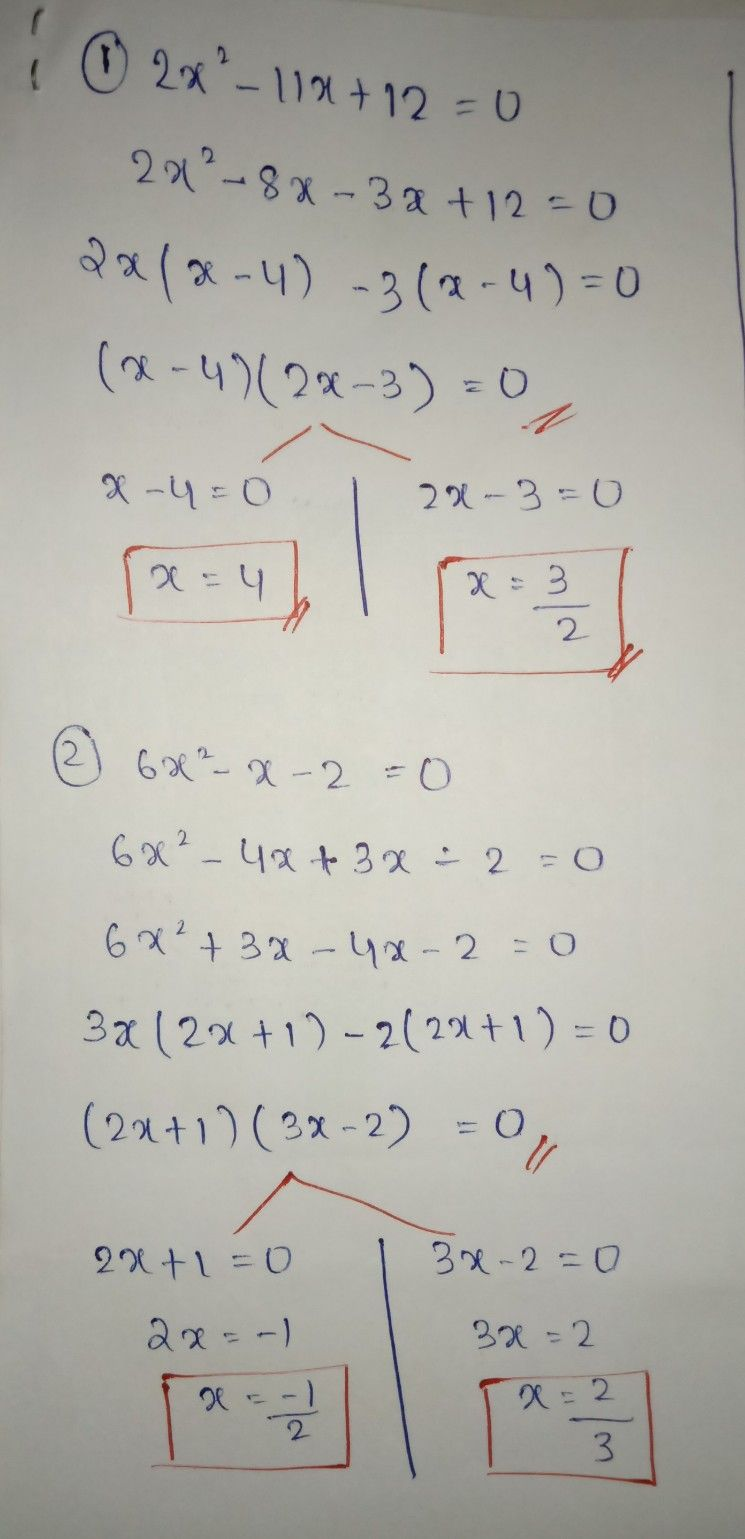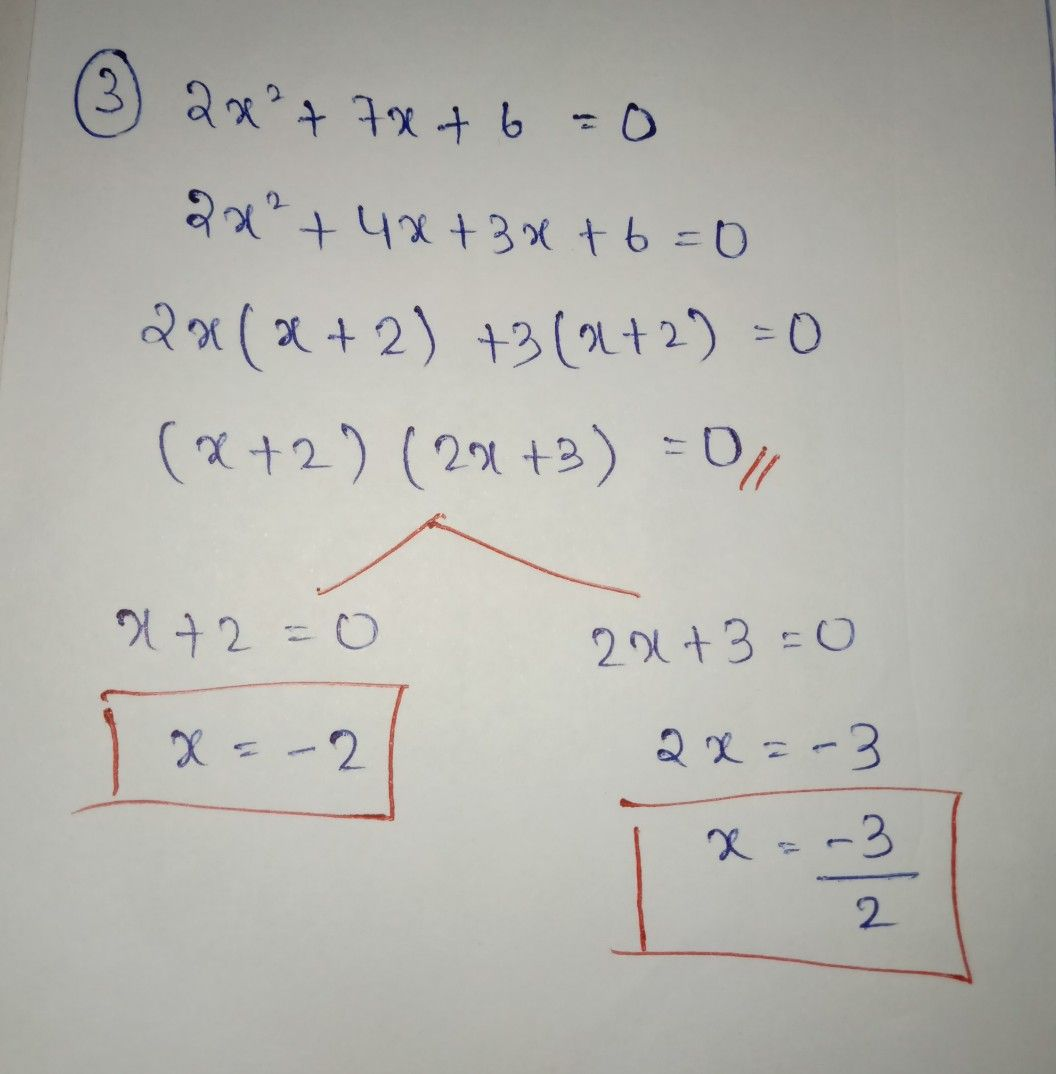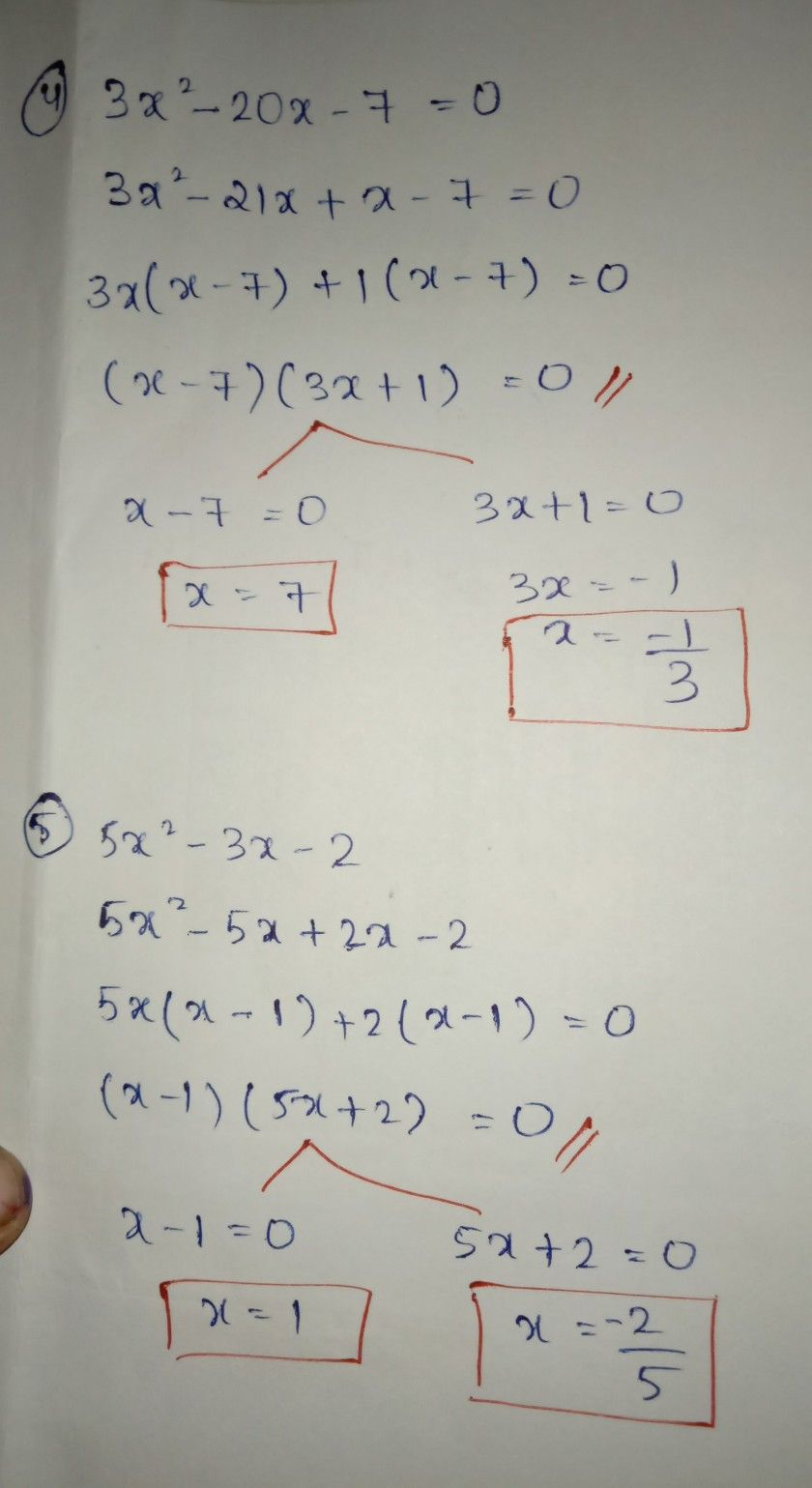Symbol
Problem$1.$ $2x^{2}-11x+12$ $1$ $2$ $6x^{2}-x-2$ $3$ $2x^{2}+7x+6$ $4.$ $3x^{2}-20x-7$ $5.$ $5x^{2}-3x-2$